## 16.3. Polar graphs

In order to create Radar graphs the module "`jpgraph_polar.php`" must first be included.

Each data point in a polar plot is represented by a tuple consisting of a radius and an angle. The polar plot itself can be either outlined or filled. In addition each point may have a standard marker (the same as for line and scatter plots).

The scale for the radius can be either linear or logarithmic.

A polar graph is created by creating an instance of `class PolarGraph`. The polar graph type inherits all the capabilities of ordinary x,y graphs, i.e they can have background images, background gradients, formatted titles, using tabbed titles etc.

There are two types of polar graphs, full 360 degree view or just 180 degree view. The figures below show the difference between these two graph types.

 Figure 16.41. A full 360 degree polar graph `(polarex0.php)`Figure 16.42. A 180 degree (half) polar graph `(polarex0-180.php)`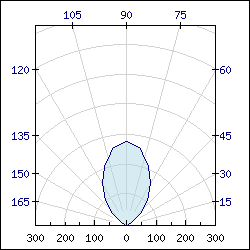The choice is controlled with the method

• `PolarGraph::SetType(\$aType)`

`\$aType` is specified with the symbolic defines

• `POLAR_360`, The default

• `POLAR_180`

As an example of using some more of the available formatting that is discussed in later sections Figure 16.43 shown a full 360 degree plot with both background gradient as well as using alpha blending for the fill of the polar plot.

Figure 16.43. A 360 polar plot with background gradient and alpha blending `(polarex7-2.php)`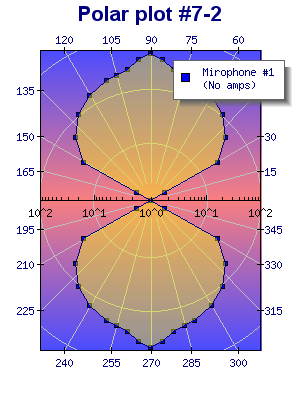### Caution

There is a bug in versions before v3.0.4 so that a rotated polar graph must always have equal margins on all side to work as expected.

By default the start angle (angle=0) is located at the 3'a clock position. By calling the method `Graph::Set90AndMargin()` it is possible to rotate the graph so that the 0-angle position is at the 6'a clock position (straight down). This is mostly used for full 360 degree graphs.

### Note

This is only available in v3.0.4 of the library and above.

By default the angle is counted counter-clockwise as is custom in most application of polar plots. However, it is also possible to have the angles counted clockwise. This is done by calling the method `Graph::SetClockwise()`.

In the following two examples we show a full 360 degree polar plots with clockwise ordering in both a standard configuration and also as a 90 degrees rotated graph.

 Figure 16.44. Clockwise polar graph `(polarclockex1.php)`Figure 16.45. Rotated clockwise polar graph `(polarclockex2.php)`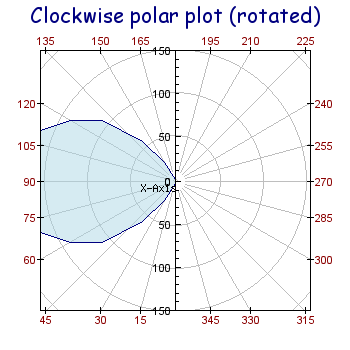### 16.3.3. Adding polar plots to a polar graph

The principle is exactly the same as for line plots but instead of an x-y coordinate each point is represented by a angle and a radius to specify its position. The data is given in the format

 ```1 ``` `\$data = array(angle1, radius1, angle2, radius2, ...)`

Creation of a single polar plot follows the now familiar structure as shown in the following code snippet

 ```1 2 3 4 5 6 7 8 9 10 11 12 13 14 15 16 17 18 19 ``` ```SetScale('log',100); // Use a full 360 degree polar graph \$graph->SetType(POLAR_360); // Create a plot with marks \$polarplot = new PolarPlot(\$data); \$polarplot->mark->SetType(MARK_SQUARE); // Add it to the graph \$graph->Add(\$polarplot); ... ?>```

Adding multiple polar plots follows the same pattern. Each polar plot is created as an instance of `class PolarPlot` and then added to the graph.

### 16.3.4. Adding plot-marks to polar plots

In exactly the same way as for line graphs it is possible to add plot marks in radar plots. The mark property is accessed through the instance variable

• `PolarPlot::mark `

(All available plot marks are listed in Appendix E)

For example to add a square marker (with the default color) the following line must be added

 ```1 ``` `\$polarplot->mark->SetType(MARK_SQUARE);`

Figure 16.46. Adding plot marks to a polar graph `(polarex7-1.php)`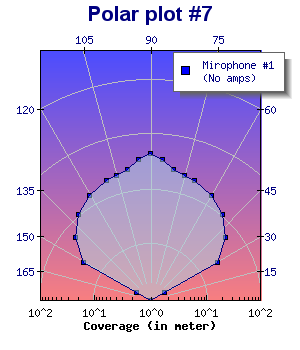### 16.3.5. Client Side Image maps

(See Chapter 10 for a full description on the usage of CSIM together with the library)

If markers are shown for the polar plot (by setting the mark property of the plot) each marker can be a hot spot in a client side image map. The target URL are as usual specified with the` SetCSIMTargets()` as the following short code snippet shows

 ```1 2 3 4 5 6 7 8 9 ``` ```mark->SetType( MARK_SQUARE ); \$polarplot->SetCSIMTargets( targets ); \$graph->Add( \$polarplot ); \$graph->StrokeCSIM(); ?>```

The radius axis can be shown in either a linear or logarithmic scale. This is controlled by a call to

• `PolarGraph::SetScale(\$aScale,\$aRadiusMax=0)`

Supported scale types for `\$aScale` are the strings "`lin`" or "`log`". The second argument is an optional manual setting of the maximum scale value for the radius.

The two examples below show the same plot in either linear or logarithmic scale for the radius

 Figure 16.47. Linear scale for radius `(polarex3-lin.php)`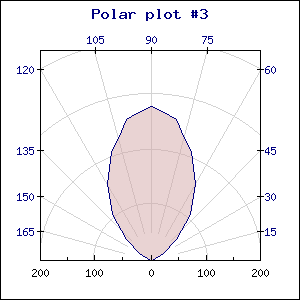Figure 16.48. Logarithmic scale for radius `(polarex3.php)`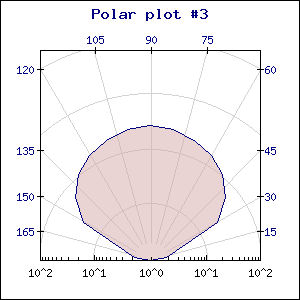Please note that the maximum values of the scales are different.

By default the scale will be auto scaled depending on the data. It is also possible to specify a manual scale by supplying an extra argument to the `SetScale()` method.

The only difference from the manual scaling with the standard x-y-graphs is that for polar graph only the maximum value is manually specified. The minimum will always be 0 for the linear scale and a scaled value of 10 (i.e 1, 0.1, 0.001 and so on) for the logarithmic scale.

### Note

The plot is always clipped to the plot area.

### 16.3.7. Adjusting the grid lines

The graph allows several formatting option for the grid lines. For polar plots there are two types of grid lines, the angle and the radius grid lines.

Grid lines format is controlled by

• `PolarAxis::ShowGrid(\$aMajor=true,\$aMinor=false,\$aAngle=true)`

Decide if the major,minor and angle grid line should be displayed

• `PolarAxis::SetGridColor(\$aMajorColor,\$aMinorColor='',\$aAngleColor='')`

Specify the color for the major,minor and angel grid

• `PolarAxis::SetAngleStep(\$aStep) `

This specifies the angle distance between two consecutive angel scale marks in degrees. By default the step size is 15 degrees.

The two example below shows a logarithmic plot with either just major grid lines or both minor and major grid lines.

 Figure 16.49. Logarithmic scale with only major grid lines `(polarex4.php)`Figure 16.50. Logarithmic scale with both major and minor grid lines `(polarex5.php)`It is possible to individually specify different fonts and colors for the angle and the radius labels.

• `PolarAxis::SetFont()`

• `PolarAxis::SetAngleFont()`

• `PolarAxis::SetColor()`

The following example specifies different color for the labels. it also shows how to add both a radial axis title as well as a tabbed title. In this example we have also chosen not to show the frame around the edge of the plot.

Figure 16.51. Different colors for labels, specifying both a tabbed title as well as a axis title `(polarex9.php)`### Note

As can be seen from the previous examples the angle labels have a degree mark "°" by default if the font used is a TTF font (the degree symbol is not available for bitmap fonts) after each label. It is possible to select if this degree mark should be displayed or not with a call to the method `PolarAxis::SetAngleDegreeMark()`.

### Note

For the radius labels all standard formatting that can be done to the x-y axis such as format string or format callbacks are supported.

### Note

A common modification for polar plots is to disable the display of the last label when using a 360 degree plot since the last label will "collide" with the plot box around the plot area. It is possible to disable the last label with a call to `Axis::HideLastTickLabel()` As can be see this has been used in some of the examples in this chapter.